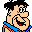Center of gravity
Firdaus from Malaysia  [3 posts]
3 years
 Currently i'm studying the movement of the fish by using roborealm software. One of the parameter that i used in this study is center of grtavity to determine the fish position. One thing that i don't understand is the formula used in generating the center of gravity. Here are the formula given from roborealm webpage, Center of Gravity The Center of Gravity or Center of Mass statistic calculates where the COG of the image lies. The COG is calculated by: COG_X = COG_X + (I*x) COG_Y = COG_Y + (I*y) Total = Total + I for each pixel where I = (R+G+B)/3 and x,y is the current pixel location. The resulting COG is then divided by the Total value: COG_X = COG_X/Total COG_Y = COG_Y/Total to result in the final x,y location of the COG Based on the formula given from the roborealm webpage, can you explain a bit more on the formula used. Thank you
Steven Gentner from United States  [268 posts] 3 years
 Firdaus, There isn't anything more to explain. That's the formula! Perhaps you can ask what part of the formula you don't understand and we can elaborate. Perhaps you don't understand the R, G, B parts? Those are the 3 RGB colors of a particular pixel. One could skip the I calculation and just use the Green channel of the pixel. Plus, there are mountains of information available via a web search. If something isn't clear try searching for that on other sites that might present the information a bit differently and in a way that makes more sense to you. STeven.Firdaus from Malaysia  [3 posts] 3 years
 Regarding the formula for Center of gravity, can you help me in understanding this equation. COG_X = COG_X + (I*x), 1.is there any differences between COG_X before = and after =. 2. What is differences between COG_X and x, 3. I = (R+G+B)/3, what is the meaning of I? and where can we get the value of R, G and B.? 4. Total = Total + I, what is Total, is it the total number of the pixel? 5. And lastly for COG_X = COG_X/ Total, if the COG_X = 100, thus it means that 100 = 100/ Total? Hopefully you can help me understanding this formula. is there any suggestion of references that I should read. Thank youSteven from United States  [1425 posts] 3 years
 1. The COG_X accumulates a value after each pixel is processed. If you are not familiar with programming languages that would explain why this formula looks strange. Its a common paradigm in programming to add to an existing value. It should be initialized to zero. 2. COG_X is the accumulating center of gravity value and x is the x coordinate of the current pixel. For a 640x480 image, this x value would range from 0 to 639 for each rown. 3. I = intensity. A pixel has 3 channels (basic image array) which when averaged can be thought of as intensity of the pixel. R = red, G = green, B = blue values of a particular pixel. If that still doesn't make sense, read up on how images are represented in terms of color values. 4. Total is the total intensity of the entire image. 5. Again, check basic programming paradigms. Its not 100 = 100 / Total but that 100 / Total is then placed back into the COG_X variable. Please just use google to find references .. there a millions of pages related to basic programming and image representation. STeven.

 This forum thread has been closed due to inactivity (more than 4 months) or number of replies (more than 50 messages). Please start a New Post and enter a new forum thread with the appropriate title.